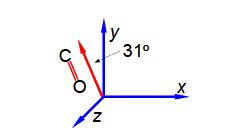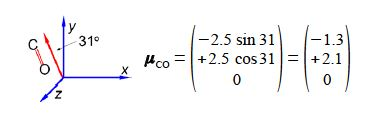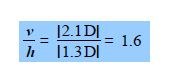# Calculate the Absorption of Light by Polar Molecules?

It is possible to arrange molecules so that the bond dipoles all point in a particular direction. Below Figure shows the alignment of carbonyl bonds in a sample. The magnitude of the carbonyl dipole moment is 2.5 D.The spectroscopic absorbance (A) of any sample is proportional to the magnitude of the component of the dipole which is parallel to the direction of polarization of the radiation used. Resolve the carbonyl dipole vector and hence calculate the ratio of the absorbances of the sample when the radiation is vertically polarized (v) to when it is horizontally polarized (h).

Solution

In this question we are given the resultant molecular dipole moment vector and we have to resolve it into its horizontal (h) and vertical (v) component dipoles.The ratio of the spectroscopic absorbances for vertical polarisation (v) to horizontal polarisation (h) is independent of the signs of the components i.e. you can’t have a negative absorbance.